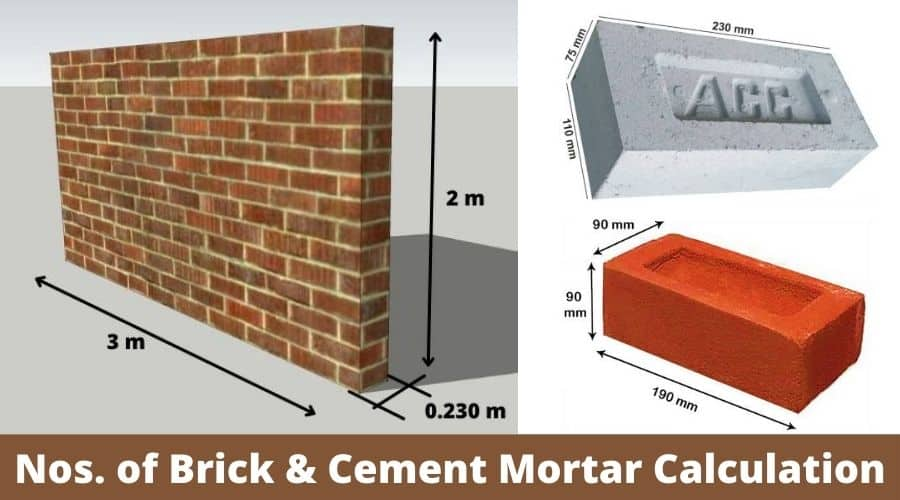# Brick Work Calculation | How to Calculate Quantity of Cement Mortar In Brickwork and Plaster6 min read

Post Contents

## What Is Brick work?

Brick work is the laying of brick in a systematic manner with the help of mortar in a number of layers. In brickwork, bricks are laid horizontally side by side and one above the other to make them strong. The structure formed by placing bricks in such a manner is called a brick masonry wall.

The brick work calculation and how to calculated quantity of cement mortar in brickwork and plaster is given below,

## Types of Bricks used in Construction

The 3 most used bricks used in building construction are as follows,

1. Red Brick

2. Fly Ash Brick

3. Hollow Brick

## Standard Size of Brick

1. Red Bricks

The standard Size of Brick in mm is 190 x 90 x 90 mm (Length x Width X Thickness)

Standard Brick Dimensions Inches is 7.48 In X 3.54 In X 3.54 In (Length x Width X Thickness)

2. Fly Ash Brick

The standard size of fly ash brick is 230 mm x 110 mm x 75 mm used in construction.

3. Hollow Brick

The standard size of hollow brick is 30x15x15 cm.

## How to Calculate Number of Brick in A wall (Brick work Calculation)

First of all, we do a brick work calculation for 1 m3 of brickwork volume.

The volume of brick work = 1 m3

Size of brick with mortar = 20 cm x 10 cm x10 cm

Standard size of brick without mortar = 19 cm x 9 cm x 9 cm

Nos of brick required in 1m3 = 1/(0.20×0.10×0.10) = 500 Nos

500 is the number of bricks required in 1 m3 with mortar. It means mortar volume is also calculated in the calculation. So we have to find out the actual numbers of bricks and the quantity of mortar.

Actual volume of Brick = 500 x (0.19×0.09×0.09) = 0.7695 m3

Volume of Mortar = 1 – 0.7695 = 0.2305 m3

Adding 10% wastage = 0.2305 + (0.2305×10/100) = 0.2305 + 0.02305 = 0.25355 m3

As this is the wet volume of mortar, the dry volume is generally 25% more than wet volume.

Add 25% Extra volume for dry volume of mortar.

= 0.25355 + (0.2355×25/100) = 0.25355 + 0.0633875 = 0.3169 m3

So, from above calculation we can say that

Nos. of Brick required for 1 m3 of brick work = 500

Mortar required for 1 m3 of brick = 0.3169 m3

## How to Calculate Quantity of Cement Mortar in Brickwork and Plaster

1. Quantity of Cement Mortar In Brick work Calculation

Let Take an example, The length of the wall = 3 m, width = 2 m, and thickness of the wall = 0.23 m.

Volume of Brickwork = 9 x 5 x 0.23 = 10.35 m3

We know that, for 1m3 brickwork = 500 Nos required

So, for 10.35 m3 of brick work = 500x 10.35 = 5175 Nos.

Also , know that for 1m3 of brickwork = 0.3169 m3 of mortar is required

So, for 10.35 m3 of brickwork = 0.3169×10.35 = 3.28 m3

Now, Let find out quantity of cement and sand in mortar,

### Mix Ratio 1:4

Consider mortar mix ration is 1:4

1 part = cement, 4 part = sand

Total part = 1+4 = 5 part

#### 1. Cement (bags)

Cement = 1/5 x (volume of mortar)

= 1/5 x 3.28

= 0.656 m3 (volume of 1 bag of cement = 0.035 m3)

= 0.656/0.035

Cement = 18.74 bags = 19 bags

#### 2. Sand (cu.ft)

Sand = 4/5 x 3.28

Sand = 2.62 m3

### For Mix Ratio (1:6)

Cement = 1 part, Sand = 6 part

Total Part = 1+6 = 7 part

#### 1. Cement (Bags)

Cement = 1/7 x 3.28

Cement = 0.46 m3 (1 bag volume of cement = 0.035 m3)

Cement = 13.14 bags

#### 2. Sand (cu. ft.)

Sand = 6/7 x 3.28

Sand = 2.18 m3

### Summary:

1. For Cement Mortar (1:4)

Cement = 19 bags , Sand = 2.62 m3 (92.52 ft3)

2. For Cement Mortar (1:6)

Cement = 13 bags, Sand = 2.18 m3 (77 ft3)

## Cement Mortar Calculation In Plasterwork

Consider a wall having length = 15 m, width = 8 m with plasterwork thickness =12 mm (0.012m)

First, Calculate Volume of Cement Plaster work

Volume of Cement Mortar in Plastering = 15x8x 0.012 = 1.44 m3

Adding 10% wastage = 1.44 + 1.44x(10/100) = 1.44 + 0.144 = 1.584 m3 (wet volume of mortar)

As this is wet volume of mortar, the dry volume is generally 25% more than wet volume.

Add 25% Extra volume for dry volume of mortar.

= 1.584 + (1.584×25/100) = 1.584 + 0.1584 = 1.74 m3

### Cement Mortar Mix Ratio = 1:4

1 part cement and 4 part sand (Total Part = (1+4) = 5 part)

#### 1. Cement (1 part)

Cement = ( 1 / 4 ) x 1.74

= 0.435 m3 (1 bag volume of cement = 0.035 m3)

= 0.435/0.035 = 12.42

Cement = 12.42 bags

#### 2. Sand (4 part)

Sand = 4/5 x 1.74

Sand = 1.392 m3

### Cement Mortar Mix Ration = 1:6

Cement part = 1 , Sand Part = 6

Cement = ( 1 / 7 ) x 1.74

= 0.24 m3 (1 bag volume of cement = 0.035 m3)

= 0.24/0.035 = 7.10

Cement = 7.10 bags

### 2. Sand (4 part)

Sand = 6/7 x 1.74

Sand = 1.49 m3

### Summary

For 1:4 Mix Cement Mortar: Cement = 12.42 bags, Sand 1.392 m3 (49.15 ft3)

For 1:4 Mix Cement Mortar: Cement = 7.10 bags, Sand 1.49 m3 (52.61 ft3)

## Watch Video: How to Calculate Number of Bricks In Brickwork

You May Also Like:

### Popular Post on Website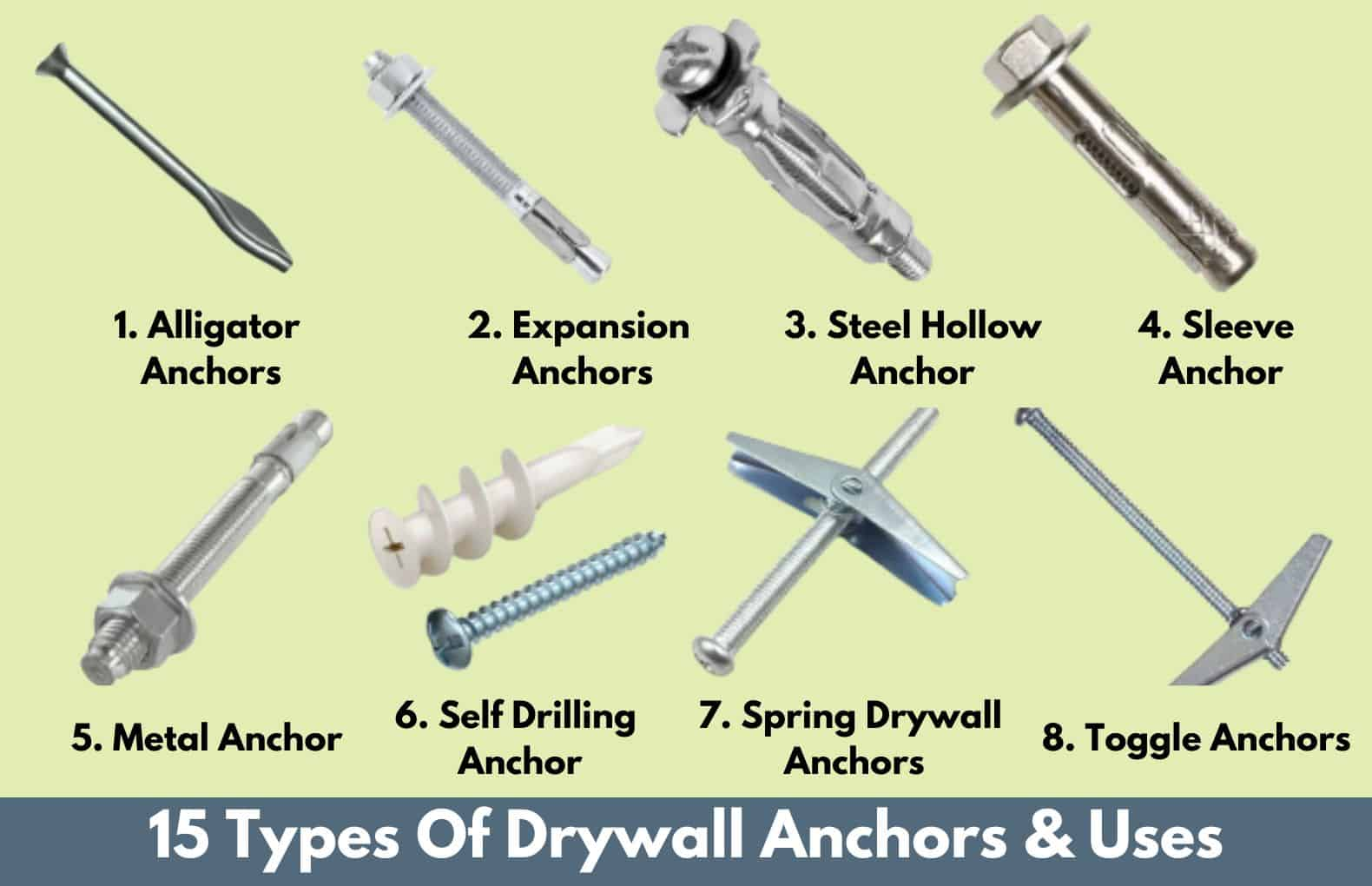#### 15 Types of Drywall Anchors | Different Types Of Wall Anchors | Dry Wall Anchors Types | Best Types of Wall Anchors For Wall#### Types Of Gutters | 22 Different Types of Gutters | Rain Gutter Downspout | Best Types of Rain Gutter For House#### 12 Types of Rugs | Different Types of Rugs With Pictures | Best Types of Rug For Living Room | Best Rug For Bedroom#### 15 Types of Mattresses | Different Types of Mattresses | Most Popular Mattress Types#### 15 Types of Fans | Different Types of Fans For Home | Ceiling Fan Type | Best Fan Types & Their Uses#### 22 Types of Drill Bits | Drill Bit Types And Uses | Different Drill Bits Name | Best Drill Bits For Cutting Work#### 40 Types Of Tables | Different Types of Tables | Best Types Of Table For Living Room | Different Kinds Of Tables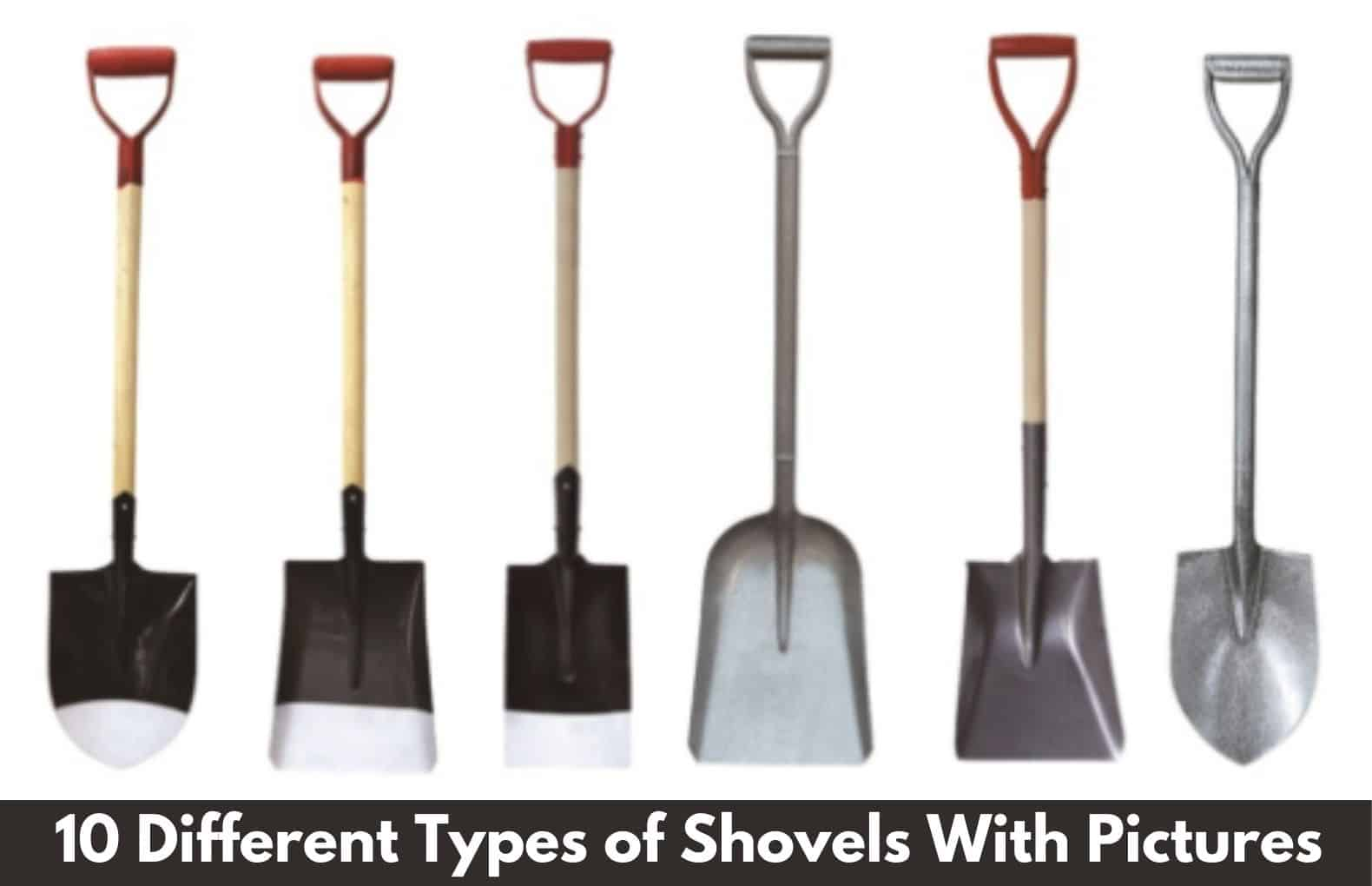#### 10 Types of Shovels | Different Types of Shovels With Pictures | Best Shovels For Digging Work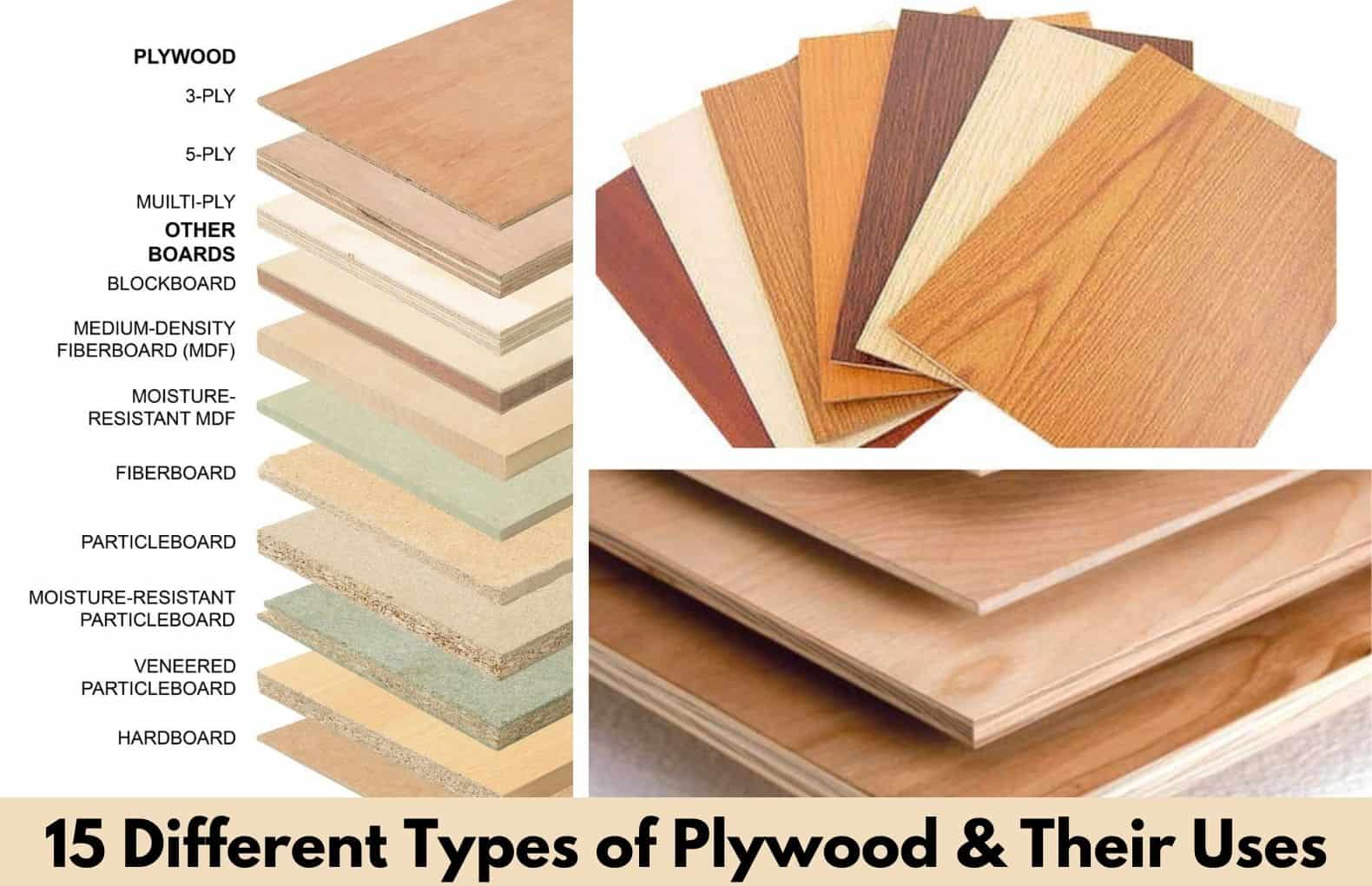#### 15 Different Types of Plywood | What Is Plywood and Their Types | 15 Best Types of Plywood for Furniture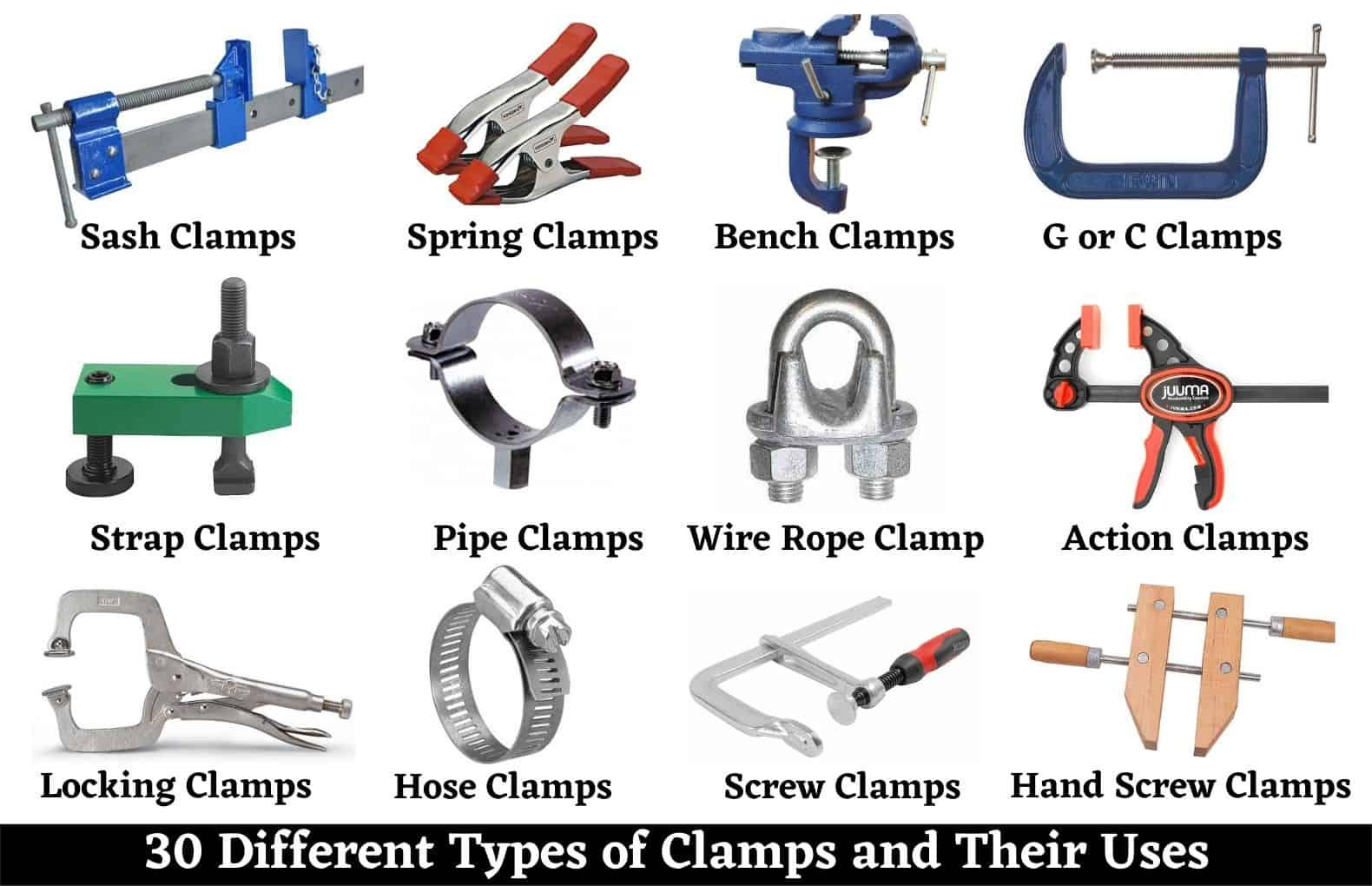#### 30 Types of Clamps and Their Uses | 30 Different Types Clamps and Uses | Best Clamps For Home Uses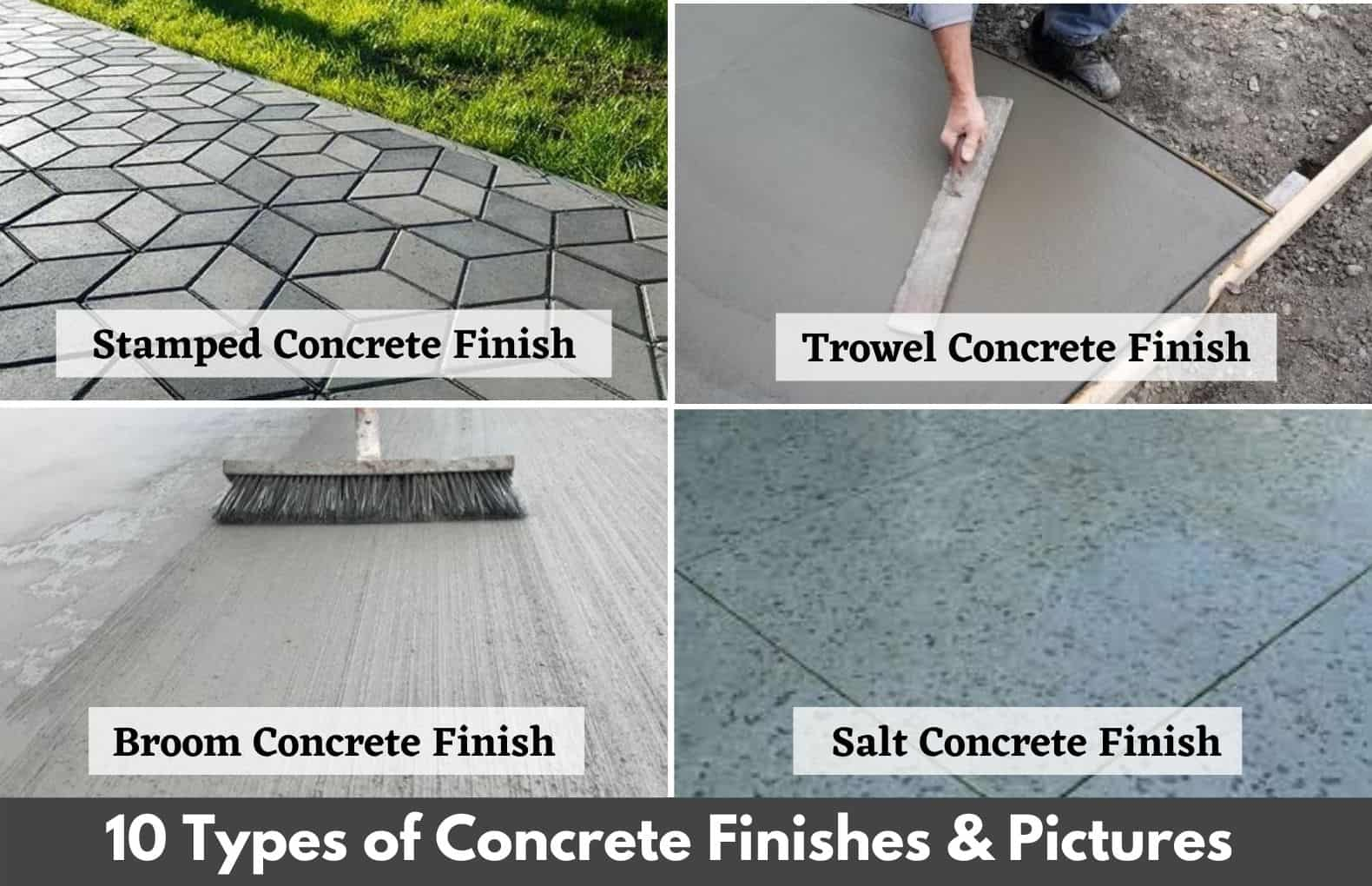#### 10 Concrete Finishes Types | 10 Best Types of Concrete Finishes | Different Concrete Finishes | Concrete Surface Finishes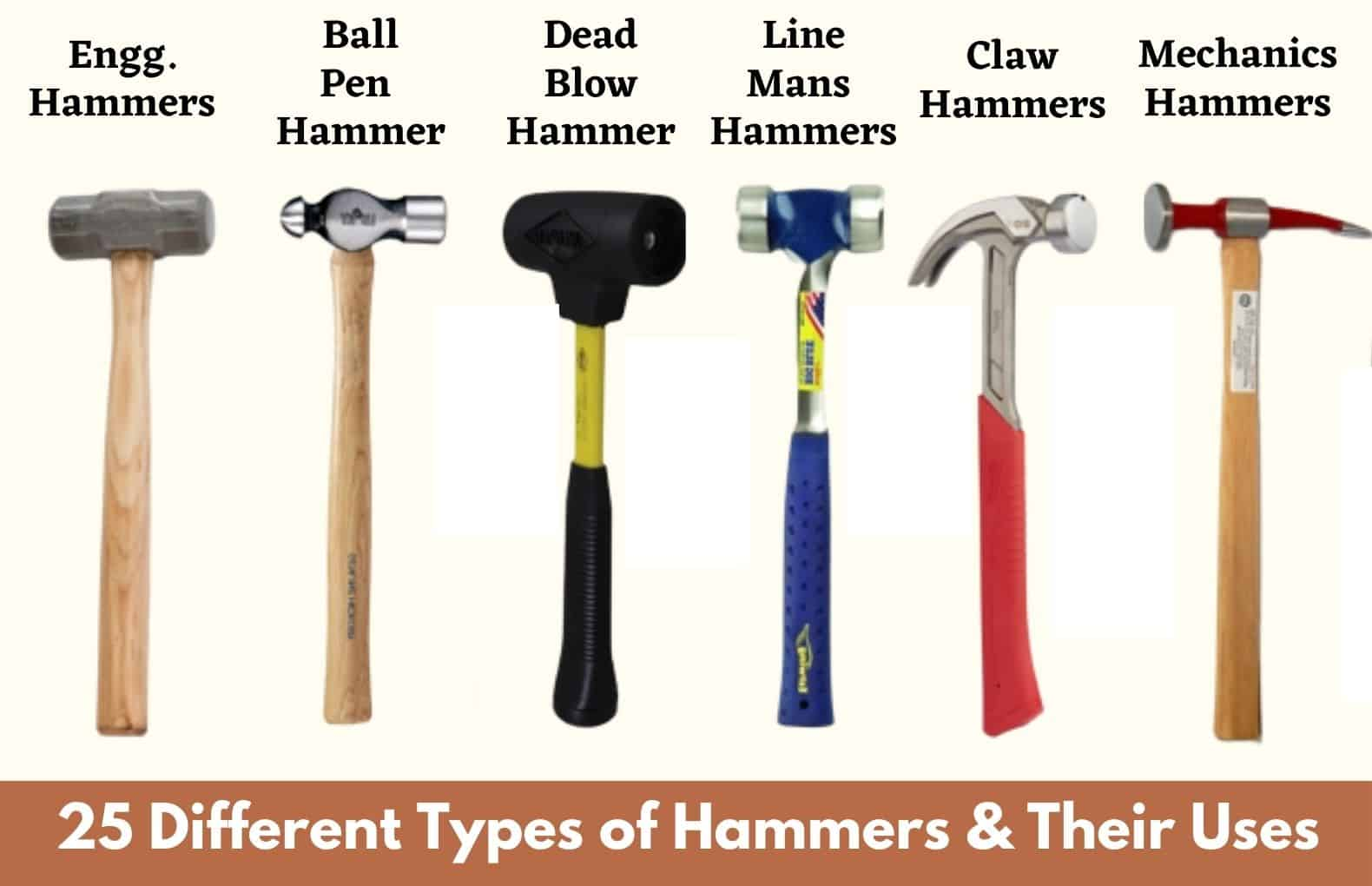#### 25 Types of Hammers | Different Types of Hammers and Their Uses | Top 25 Hammers Types#### 10 Different Types of Ladders and Their Uses | Best Types of Ladders | Material Used For Ladders#### 17 Types Of Contractors | What Is Contractor | 17 Different Types of Contractors | List of Contractors﻿
x

### Related Posts

Setting Time of Cement | Initial and Final Setting Time of Cement Test | Initial Setting Time of Cement | Final Setting Time of Cement
What Is Setting Time of Cement? Initial setting time gives an idea about how fast cement can start losing its plasticity and the final settin...
7 Brick Test | Tests on Bricks | Testing of Bricks | Brick Quality Check Test
7 Different Types of Tests on Bricks Brick is the oldest and most extensively used construction material. Bricks are popular construction mat...
Which Cement Is Best for House Construction | Which Cement Is Best | Best Cement In India | Best Cement for Slab | Best Cement For Roof
Which Cement Is Best for House Construction Which Cement is Best for House Construction is a common question asked by many people who are thi...